# 联合索引在B+树上的存储结构及数据查找方式

baojunzh

0收藏

## 引言

• 联合索引在B+树上的存储结构
• 联合索引的查找方式
• 为什么会有最左前缀匹配原则

## 联合索引的存储结构

(https://segmentfault.com/q/1010000017579884)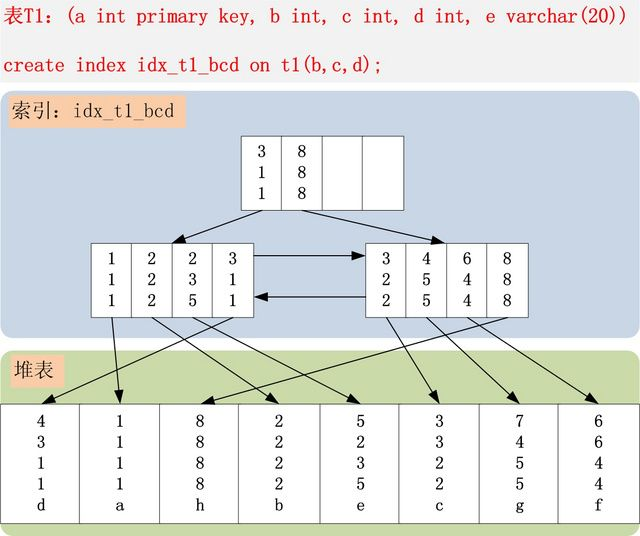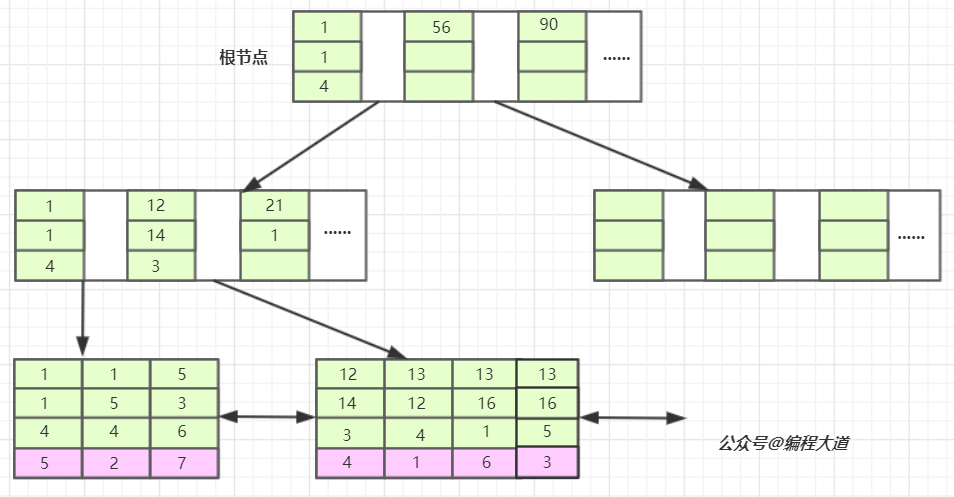bcd联合索引在B+树上的结构图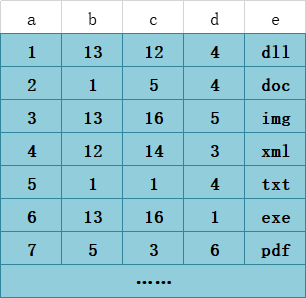T1表

## 联合索引的查找方式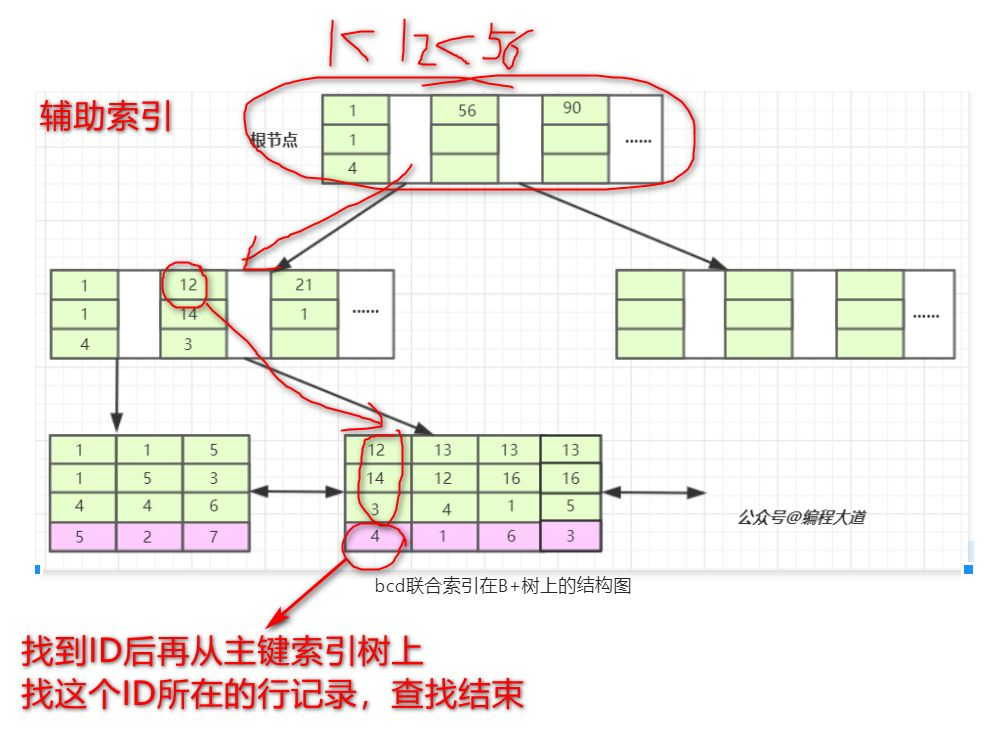## 最左前缀匹配原则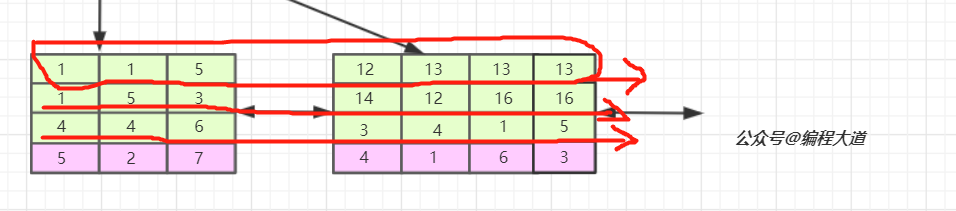M

毛 不易   178********

马 化腾   183********

马 云     188********

Z

张 杰     189********

张 靓颖   138********

张 艺兴   176********

### 实践

``````select * from T1 where b = 12 and c = 14 and d = 3;-- 全值索引匹配 三列都用到
select * from T1 where b = 12 and c = 14 and e = 'xml';-- 应用到两列索引
select * from T1 where b = 12 and e = 'xml';-- 应用到一列索引
select * from T1 where b = 12  and c >= 14 and e = 'xml';-- 应用到一列索引及索引条件下推优化
select * from T1 where b = 12  and d = 3;-- 应用到一列索引  因为不能跨列使用索引 没有c列 连不上
select * from T1 where c = 14  and d = 3;-- 无法应用索引，违背最左匹配原则``````

## 后记帖子
视频
声望
粉丝
最近发布
社区精华内容